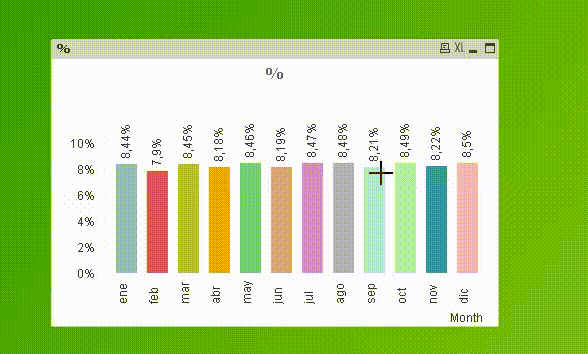# QlikView App Dev

Discussion Board for collaboration related to QlikView App Development.

Announcements
Now Live: Qlik Sense SaaS Simplified Authoring – Analytics Creation for Everyone: READ DETAILS
cancel
Showing results for
Did you mean:Not applicable

## Showing percentages in qlikview

Hi All

I'm using the below expression to calculate the percentage values

=sum({<my={'1'}>}sales)/sum({<my={'1'}>}total(sales)) *100

and my output values are

9.683284295

8.614611669

i want it to be rounded to two decimals and a %age symbol ate the end

9.68 %

8.61% i want like this

and for the below expression

=round(sum(sales)/sum(total(sales)100,0.01)

also i want the values as same rounded to two decimals ans a %age symbol

7 RepliesSpecialist

Hi,

:Master

Hi John,

Don't bother multiplying by 100. take that out so you have a decimal fraction then format the result by wrapping it in the num function like this:

=num(YourExpressionHere,'0.00%')

cheers

AndewMVP

=Num(Round(sum({<my={'1'}>}sales)/sum({<my={'1'}>}total sales),0.0001),'0.00%')

or to only format with two decimal places (not changing the actual value):

=Num(sum({<my={'1'}>}sales)/sum({<my={'1'}>}total sales),'0.00%')

hope this helps

regards

MarcoNot applicable
Author

Hi Macro

Can you explain me how it is working

the logic behind the

,0.0001),'0.00%')

and

One of the toughest requirement is, comparing the top n values in current period to the same top n values in the previous period and pre previous period can you please help us in this

The easiest way to achive and the logical way.MVP

Hi,

Round( xxx , 0.0001)   rounds to the nearest multiple of 0.01%, while Num( xxx , '0.00%') formats a number as percentage with 2 decimal places.

hope this helps

regards

MarcoSpecialist III

Hi John,

Here is the way,

Right Click on your Chart > Chart Properties > Select your expression > Select Checkbox "Relative" > Apply > OK.

Kind regards,

Ishfaque AhmedContributor

I was struggling to get the correct percentage but your comment suggesting "not to multiply by 100" solved it. Thanks!